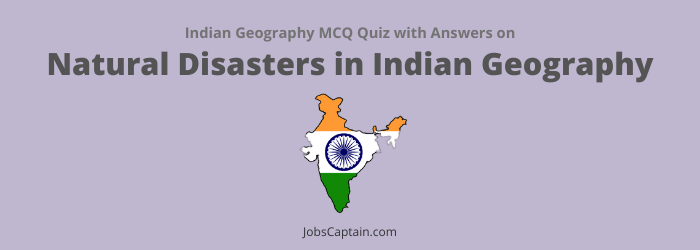# MCQ on Natural Disaster with Answers PDFQuestion 1. Tsunami Warning Centre in India is located in __________.

(A) Port Blair

(C) Mumbai

(D) Chennai

Question 2. Indian Meteorological Department is established at __________.

(A) Pune

(B) Jodhpur

(C) Nagpur

(D) New Delhi

(D) New Delhi

Question 3. Which of the following coasts of India was worst affected by 2004 Tsunami?

(A) Northern Circars Coast

(B) Coromandel Coast

(C) Malabar Coast

(D) Malabar Coast

(B) Coromandel Coast

Question 4. Which of the following coastal areas of India was affected by ‘Hudhud Cyclone’?

(A) Bengal coast

(B) Chennai coast

(C) Kerala coast

Question 5. Cyclones are more frequent in the coastal areas of Bay of Bengal, because __________.

(A) The conical shape of Bay of Bengal funnels cyclones Northwards when they are formed in the sea

(B) Long chain of isand of Andaman and Nicobar acts as launching pad for cyclones

(C) Water in the Bay of Bengal has chemicals which help in formation of cyclones

(D) High temperatures in the Bay of Bengal

(D) High temperatures in the Bay of Bengal

Question 6. Which among the following states faces maximum natural disaster?

(A) Gujarat

(B) Bihar

(C) Odisha

(C) Odisha

Question 7. The first Disaster Management Training Institution of the country is being established at __________.

(B) Latur (Maharashtra)

(C) Bangalore (Karnataka)

(B) Latur (Maharashtra)

Question 8. Which one of the following areas of India does not come under the zone of high seismic intensity?

(B) Kutch

(C) Karnataka Plateau

(D) Uttarakhand

(C) Karnataka Plateau

Question 9. India has been divided into how many Seismic Risk Zones?

(A) 7

(B) 4

(C) 6

(D) 5

(B) 4

Question 10. Which one of the following is not correctly matched?

 Cities Seismic Zones (A) Lakshadweep III (B) Hyderabad I (C) Srinagar V (D) Chennai II

Question 11. The most flood-prone state of India is __________.

(B) Bihar

(D) Assam

(B) Bihar

Question 12. Match List-I (Natural Hazards) with List-II (Regions) and select the correct answer using the code given below the lists.

 List-I (Natural Hazards) List-II (Regions) (a) Floods 1. Himalayan Foothill Zone (b) Earthquakes 2. Jharkhand and Northern Odisha (c) Droughts 3. Plains of Uttar Pradesh and Bihar (d) Cyclones 4. Mid-Eastern India
 (a) (b) (c) (d) (A) 3 1 2 4 (B) 3 1 4 2 (C) 2 3 1 4 (D) 4 2 3 1
(B) 3 1 4 2

Question 13. Among the following area of Uttar Pradesh which is maximum flood affected?

(A) Northern area

(B) Middle area

(C) Eastern area

(D) Western area

(C) Eastern area

Question 14. Given below are two statements. One is labelled as Assertion (A) and other as Reason (R).

• Assertion (A): The frequency of land slides has increased in the Himalayas.
• Reason (R): There has been large scale mining in the Himalayas in recent years.

(A) (A) is false, but (R) is true

(B) (A) is true, but (R) is false

(C) Both (A) and (R) are true, but (R) is not the correct explanation of (A)

(D) Both (A) and (R) are true and (R) is the correct explanation of (A)

(D) Both (A) and (R) are true and (R) is the correct explanation of (A)

Question 15.

•  Assertion (A): East coast is most prone to cyclones than Western coast.
• Reason (R): Easter coast of India lies in the zone of North-East trade winds.

In the context of above statements, which of the following is correct?

(A) (A) is false, but (R) is true

(B) (A) is true, but (R) is false

(C) Both (A) and (R) are true, but (R) is not the correct explanation of (A)

(D) Both (A) and (R) are true and (R) is the correct explanation of (A)

(C) Both (A) and (R) are true, but (R) is not the correct explanation of (A)

Question 16.

• Assertion (A): The Koyna region of Maharashtra is likely to become more earthquake prone in near future.
• Reason (R): The Koyna dam is located on an old fault-plane which may get activated more frequently with changes in water-level in Koyna reservoir.

Select the correct answer using the code given below.

(A) (A) is false, but (R) is true

(B) (A) is true, but (R) is false

(C) Both (A) and (R) are true, but (R) is not the correct explanation of (A)

(D) Both (A) and (R) are true and (R) is the correct explanation of (A)

(D) Both (A) and (R) are true and (R) is the correct explanation of (A)

Question 17.

• Assertion (A): The frequency of floods in North Indian plains has increased during the last couple of decades.
• Reason (R): There has been reduction in the depth of river valleys due to deposition of silt.

(A) (A) is false, but (R) is true

(B) (A) is true, but (R) is false

(C) Both (A) and (R) are true, but (R) is not the correct explanation of (A)

(D) Both (A) and (R) are true and (R) is the correct explanation of (A)